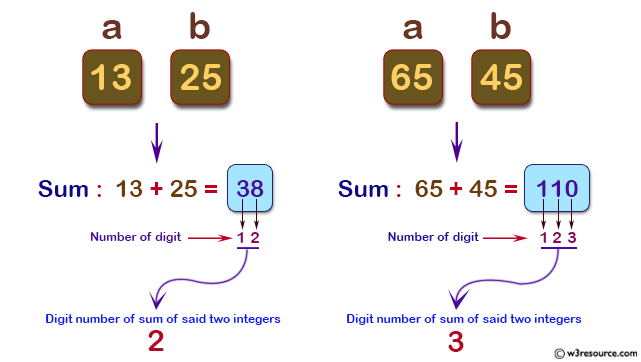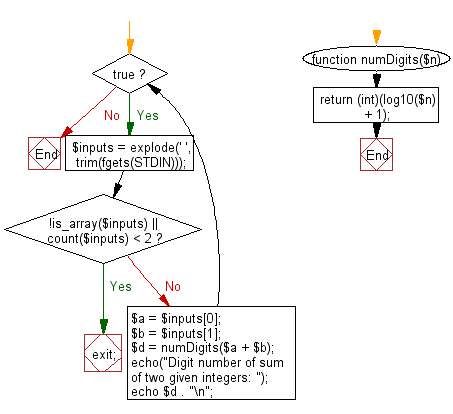﻿ PHP Exercise: Compute the digit number of sum of two given integers - w3resource

# PHP Exercises: Compute the digit number of sum of two given integers

## PHP: Exercise-47 with Solution

Write a PHP program to compute the digit number of sum of two given integers.

Input:
Each test case consists of two non-negative integers x and y which are separated by a space in a line.
0 ≤ x, y ≤ 1,000,000

Pictorial Presentation:Sample Solution: -

PHP Code:

``````<?php
while(true) {
\$inputs = explode(' ', trim(fgets(STDIN)));
if (!is_array(\$inputs) || count(\$inputs) < 2) {
exit;
}
\$a = \$inputs;
\$b = \$inputs;
\$d = numDigits(\$a + \$b);
echo("Digit number of sum of two given integers: ");
echo \$d . "\n";
}
function numDigits(\$n) {
return (int)(log10(\$n) + 1);
}
?>
```
```

Sample Output:

```Digit number of sum of two given integers: 2
```

Flowchart:PHP Code Editor:

Have another way to solve this solution? Contribute your code (and comments) through Disqus.

What is the difficulty level of this exercise?

Test your Programming skills with w3resource's quiz.

﻿

## PHP: Tips of the Day

PHP: Correct file permissions for WordPress

When you setup WP you (the webserver) may need write access to the files. So the access rights may need to be loose.

```chown www-data:www-data  -R * # Let Apache be owner
find . -type d -exec chmod 755 {} \;  # Change directory permissions rwxr-xr-x
find . -type f -exec chmod 644 {} \;  # Change file permissions rw-r--r-
```

After the setup you should tighten the access rights, according to Hardening WordPress all files except for wp-content should be writable by your user account only. wp-content must be writable by www-data too.

```chown <username>:<username>  -R * # Let your useraccount be owner
chown www-data:www-data wp-content # Let apache be owner of wp-content
```

Maybe you want to change the contents in wp-content later on. In this case you could

• temporarily change to the user to www-data with su,
• give wp-content group write access 775 and join the group www-data or
• give your user the access rights to the folder using ACLs.

Whatever you do, make sure the files have rw permissions for www-data.

Ref : https://bit.ly/3hcrTkL

We are closing our Disqus commenting system for some maintenanace issues. You may write to us at reach[at]yahoo[dot]com or visit us at Facebook